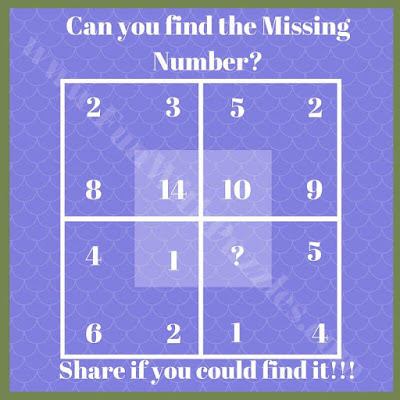## Thursday, May 21, 2020

A few days back we have published many different types of Math Brain Teasers. Here are more Mathematical brain teasers to tickle your mind. Logical reasoning is also required to solve these Maths puzzles as numbers given in each of the group are related to reaching others with the Logic and Maths.
In these Maths Puzzles Brain Teasers, four sets of numbers are given. These numbers are related to each other Mathematical and Logical by a sequence or pattern. Your challenge is to find these relationships in each of these Math Puzzles Brain Teasers and then solve it for the missing number which will replace the question mark.
Answers of these Maths Brain Teasers are not given as of now. Do try these puzzles and try to find the solution for each of these brain teasers. Once you are able to solve it do write your answers along with your explanation in the comments.1. Can you find the missing Number?2. Can you calculate the value of Question Mark?3. Find the Number which will replace the Question Mark.4. Can you solve it for missing Number?5. Can you find the missing number which replaces the question mark?

List of Similar Maths Puzzles Brain Teasers
Do not miss to checkout following Math Brain Teasers

1. Out of Box Thinking Fun Brain Teasers: This contains more than 20 Brain Teasers which is a mix of Mathematical and Logical Reasoning.

2. Brainteasers to Test Your Mental Sharpness: These are tough parking puzzles brain teasers in which one has to tell the number on the parking space by doing mathematical calculations and logical reasoning.

3. Fun Brain Teasers: There are more than 25 Fun Brain Teasers which includes all types of puzzles.

4. Cool Abbreviation Brain Teasers for Kids with Answers: Do checkout these cool abbreviation fun brain teasers.

1.2.1.